# Balance puzzle facts for kids

Kids Encyclopedia Facts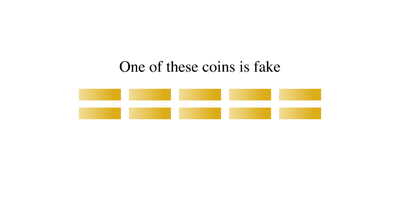An animation of a solution to the a false coin problem involving ten coins. In this example, the false coin is lighter than the others.

A balance puzzle or weighing puzzle is a logic puzzle about balancing items—often coins—to determine which holds a different value, by using balance scales a limited number of times. These differ from puzzles that assign weights to items, in that only the relative mass of these items is relevant.

Known Goal Maximum Coins for n weighings Number of Weighings for c coins
Whether target coin is lighter or heavier than others Identify coin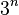$3^n$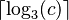$\lceil\log_3(c)\rceil$
Target coin is different from others Identify coin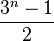$\frac{3^n-1}2$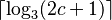$\lceil\log_3(2c+1)\rceil$
Target coin is different from others, or all coins are the same Identify if unique coin exists, and whether it is lighter or heavier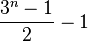$\frac{3^n-1}2-1$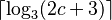$\lceil\log_3(2c+3)\rceil$

For example, in detecting a dissimilar coin in three weighings (n = 3), the maximum number of coins that can be analyzed is 33 − 12 = 13. Note that with 3 weighs and 13 coins, it is not always possible to determine the identity of the last coin (whether it is heavier or lighter than the rest), but merely that the coin is different. In general, with n weighs, you can determine the identity of a coin if you have 3n − 12 - 1 or less coins. In the case n = 3, you can truly discover the identity of the different coin out of 12 coins.

## Nine-coin problem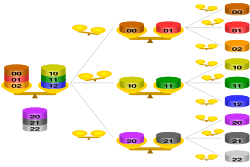Solution to the balance puzzle for 9 coins in 2 weighings, where the odd coin is lighter than the others – if the odd coin were heavier than the others, the upper two branches in each weighing decision are swapped

A well-known example has up to nine items, say coins (or balls), that are identical in weight except one, which is lighter than the others—a counterfeit (an oddball). The difference is perceptible only by weighing them on scale—but only the coins themselves can be weighed. How can one isolate the counterfeit coin with only two weighings?

### Solution

To find a solution, we first consider the maximum number of items from which one can find the lighter one in just one weighing. The maximum number possible is three. To find the lighter one, we can compare any two coins, leaving the third out. If the two coins weigh the same, then the lighter coin must be one of those not on the balance. Otherwise, it is the one indicated as lighter by the balance.

Now, imagine the nine coins in three stacks of three coins each. In one move we can find which of the three stacks is lighter (i.e. the one containing the lighter coin). It then takes only one more move to identify the light coin from within that lighter stack. So in two weighings, we can find a single light coin from a set of 3 × 3 = 9.

By extension, it would take only three weighings to find the odd light coin among 27 coins, and four weighings to find it from 81 coins.

## Twelve-coin problem

A more complex version has twelve coins, eleven or twelve of which are identical. If one is different, we don't know whether it is heavier or lighter than the others. This time the balance may be used three times to determine if there is a unique coin—and if there is, to isolate it and determine its weight relative to the others. (This puzzle and its solution first appeared in an article in 1945.) The problem has a simpler variant with three coins in two weighings, and a more complex variant with 39 coins in four weighings.

### Solution

This problem has more than one solution. One is easily scalable to a higher number of coins by using base-three numbering: labeling each coin with a different number of three digits in base three, and positioning at the n-th weighings all the coins that are labeled with the n-th digit identical to the label of the plate (with three plates, one on each side of the scale and one off the scale). Other step-by-step procedures are similar to the following. It is less straightforward for this problem, and the second and third weighings depend on what has happened previously, although that need not be the case (see below).

• Four coins are put on each side. There are two possibilities:
1. One side is heavier than the other. If this is the case, remove three coins from the heavier side, move three coins from the lighter side to the heavier side, and place three coins that were not weighed the first time on the lighter side. (Remember which coins are which.) There are three possibilities:
1.a) The same side that was heavier the first time is still heavier. This means that either the coin that stayed there is heavier or that the coin that stayed on the lighter side is lighter. Balancing one of these against one of the other ten coins reveals which of these is true, thus solving the puzzle.
1.b) The side that was heavier the first time is lighter the second time. This means that one of the three coins that went from the lighter side to the heavier side is the light coin. For the third attempt, weigh two of these coins against each other: if one is lighter, it is the unique coin; if they balance, the third coin is the light one.
1.c) Both sides are even. This means that one of the three coins that was removed from the heavier side is the heavy coin. For the third attempt, weigh two of these coins against each other: if one is heavier, it is the unique coin; if they balance, the third coin is the heavy one.
2. Both sides are even. If this is the case, all eight coins are identical and can be set aside. Take the four remaining coins and place three on one side of the balance. Place 3 of the 8 identical coins on the other side. There are three possibilities:
2.a) The three remaining coins are lighter. In this case you now know that one of those three coins is the odd one out and that it is lighter. Take two of those three coins and weigh them against each other. If the balance tips then the lighter coin is the odd one out. If the two coins balance then the third coin not on the balance is the odd one out and it is lighter.
2.b) The three remaining coins are heavier. In this case you now know that one of those three coins is the odd one out and that it is heavier. Take two of those three coins and weigh them against each other. If the balance tips then the heavier coin is the odd one out. If the two coins balance then the third coin not on the balance is the odd one out and it is heavier.
2.c) The three remaining coins balance. In this case you just need to weigh the remaining coin against any of the other 11 coins and this tells you whether it is heavier, lighter, or the same.

## Variations

Given a population of 13 coins in which it is known that 1 of the 13 is different (mass) from the rest, it is simple to determine which coin it is with a balance and 3 tests as follows:

1) Subdivide the coins in to 2 groups of 4 coins and a third group with the remaining 5 coins.
2) Test 1, Test the 2 groups of 4 coins against each other:
a. If the coins balance, the odd coin is in the population of 5 and proceed to test 2a.
b. The odd coin is among the population of 8 coins, proceed in the same way as in the 12 coins problem.
3) Test 2a, Test 3 of the coins from the group of 5 coins against any 3 coins from the population of 8 coins:
a. If the 3 coins balance, then the odd coin is among the remaining population of 2 coins. Test one of the 2 coins against any other coin; if they balance, the odd coin is the last untested coin, if they do not balance, the odd coin is the current test coin.
b. If the 3 coins do not balance, then the odd coin is from this population of 3 coins. Pay attention to the direction of the balance swing (up means the odd coin is light, down means it is heavy). Remove one of the 3 coins, move another to the other side of the balance (remove all other coins from balance). If the balance evens out, the odd coin is the coin that was removed. If the balance switches direction, the odd coin is the one that was moved to the other side, otherwise, the odd coin is the coin that remained in place.

## Generalizations

The generalization of this problem is described in Chudnov.

Let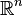$\mathbb{R}^n$ be the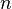$n$-dimensional Euclidean space,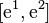$[\mathrm{e}^1, \mathrm{e}^2]$ be the inner product of vectors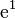$\mathrm{e}^1$ and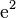$\mathrm{e}^2$ from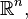$\mathbb{R}^n,$ For vectors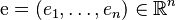$\mathrm{e} = (e_1, \dots,e_n) \in \mathbb{R}^n$ and subsets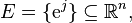$E = \{\mathrm{e}^j\} \subseteq \mathbb{R}^n,$ the operations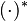$(\cdot)^{*}$ and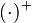$(\cdot)^{+}$ are defined, respectively, as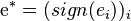$\mathrm{e}^{*} = (sign(e_i))_i$ ;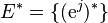$E^{*} = \{(\mathrm{e}^j) ^{*}\}$,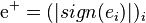$\mathrm{e}^{+} = (|sign(e_i)|)_i$,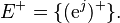$E^{+} = \{(\mathrm{e}^j)^{+}\}.$ By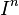$I^n$ we shall denote the discrete [−1; 1]-cube in$\mathbb{R}^n$; i.e., the set of all sequences of length$n$ over the alphabet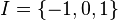$I = \{ -1,0, 1 \}$ The set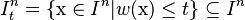$I^n_t = \{\mathrm{x} \in I^n |w(\mathrm{x}) \le t \} \subseteq I^n$ is the discrete ball of radius$t$ (in the Hamming metric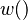$w()$ ) with centre at the point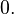$\mathrm{0}.$ Relative weights of$n$ objects are given by a vector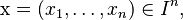$\mathrm{x} = (x_1, \dots, x_n) \in I^n ,$ which defines the configurations of weights of the objects: the$i$th object has standard weight if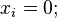$x_i = 0;$ the weight of the$i$th object is greater (smaller) by a constant (unknown) value if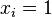$x_i = 1$ (respectively,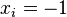$x_i = -1$). The vector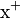$\mathrm{x}^{+}$ characterizes the types of objects: the standard type, the non-standard type (i.e., configurations of types), and it does not contain information about relative weights of non-standard objects.

A weighing (a check) is given by a vector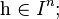$\mathrm{h} \in I^n ;$ the result of a weighing for a situation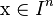$\mathrm{x} \in I^n$ is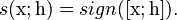$s(\mathrm{x}; \mathrm{h}) = sign([\mathrm{x}; \mathrm{h}]).$ The weighing given by a vector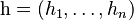$\mathrm{h} = (h_1, \dots, h_n)$ has the following interpretation: for a given check the$i$th object participates in the weighing if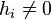$h_i \ne 0$; it is put on the left balance pan if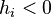$h_i < 0$ and is put on the right pan if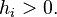$h_i > 0.$ For each weighing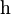$\mathrm{h}$, both pans should contain the same number of objects: if on some pan the number of objects is smaller than as it should be, then it receives some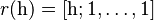$r(\mathrm{h}) = [\mathrm{h}; 1 ,\dots, 1]$ reference objects. The result of a weighing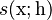$s(\mathrm{x}; \mathrm{h})$ describes the following cases: the balance if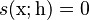$s(\mathrm{x}; \mathrm{h}) = 0$, the left pan outweighs the right one if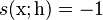$s(\mathrm{x}; \mathrm{h}) = -1$, and the right pan outweighs the left one if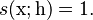$s(\mathrm{x}; \mathrm{h}) = 1.$ The incompleteness of initial information about the distribution of weights of a group of objects is characterized by the set of admissible distributions of weights of objects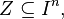$Z \subseteq I^n,$ which is also called the set of admissible situations, the elements of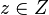$z \in Z$ are called admissible situations.

Each weighing$\mathrm{h}$ induces the partition of the set$I^n$ by the plane (hyperplane )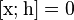$[\mathrm{x}; \mathrm{h}] = 0$ into three parts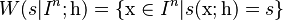$W(s|I^n ; \mathrm{h}) = \{\mathrm{x} \in I^n |s(\mathrm{x}; \mathrm{h}) = s\}$,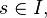$s \in I,$ and defines the corresponding partition of the set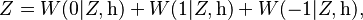$Z = W(0|Z, \mathrm{h}) + W(1|Z, \mathrm{h}) + W(-1|Z, \mathrm{h}),$ where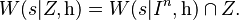$W(s|Z, \mathrm{h}) = W(s|I^n , \mathrm{h}) \cap Z.$

Definition 1. A weighing algorithm (WA)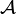$\mathcal{A}$ of length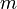$m$ is a sequence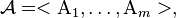$\mathcal{A} = < \mathrm {A}_1, \dots, \mathrm {A}_m >,$ where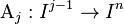$\mathrm {A}_j : I^{j-1} \to I^n$ is the function determining the check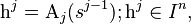$\mathrm{h}^j = \mathrm{A}_j(s^{j-1}); \mathrm{h}^j \in I^n,$ at each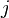$j$th step,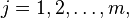$j = 1, 2, \dots, m,$ of the algorithm from the results of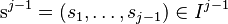$\mathrm{s}^{j-1} = (s_1, \dots, s_{j-1}) \in I^{j-1}$ weighings at the previous steps (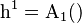$\mathrm{h}^1 = \mathrm {A}_1()$ is a given initial check).

Let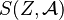$S(Z, \mathcal{A})$ be the set of all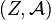$(Z, \mathcal{A})$-syndromes and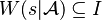$W(s|\mathcal{A}) \subseteq I$ be the set of situations with the same syndrome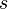$s$; i.e.,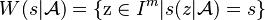$W(s|\mathcal{A}) = \{\mathrm{z} \in I^m |s(z|\mathcal{A}) = s \}$;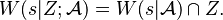$W(s|Z; \mathcal{A}) =W(s|\mathcal{A}) \cap Z.$

Definition 2. A WA$\mathcal{A}$ is said to: a) identify the situations in a set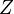$Z$ if the condition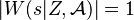$|W(s|Z, \mathcal{A}) | = 1$ is satisfied for all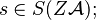$s \in S(Z\mathcal{A});$ b) identify the types of objects in a set$Z$ if the condition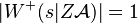$|W^{+}(s|Z\mathcal{A})| = 1$ is satisfied for all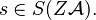$s \in S(Z \mathcal{A}).$

It is proved in that for so-called suitable sets$Z$ an algorithm of identification the types identifies also the situations in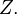$Z.$

As an example the perfect dynamic (two-cascade) algorithms with parameters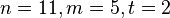$n = 11, m = 5, t = 2$ are constructed in which correspond to the parameters of the perfect ternary Golay code (Virtakallio-Golay code). At the same time, it is established that a static WA (i.e. weighting code) with the same parameters does not exist.

Each of these algorithms using 5 weighings finds among 11 coins up to two counterfeit coins which could be heavier or lighter than real coins by the same value. In this case the uncertainty domain (the set of admissible situations) contains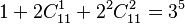$1 + 2 C_{11} ^ 1 + 2 ^ 2 C_{11} ^ 2=3^5$ situations, i.e. the constructed WA lies on the Hamming bound for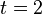$t=2$ and in this sense is perfect.

To date it is not known whether there are other perfect WA that identify the situations in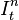$I_t^n$ for some values of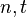$n, t$. Moreover, it is not known whether for some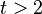$t> 2$ there exist solutions for the equation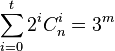$\sum_ {i = 0}^t 2^iC_n^i = 3^m$ (corresponding to the Hamming bound for ternary codes) which is, obviously, necessary for the existence of a perfect WA. It is only known that for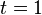$t = 1$ there are no perfect WA, and for$t = 2$ this equation has the unique nontrivial solution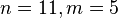$n=11, m=5$ which determines the parameters of the constructed perfect WA.

## Original parallel weighings puzzle

Konstantin Knop invented this puzzle. There are N indistinguishable coins, one of which is fake (it is not known whether it is heavier or lighter than the genuine coins, which all weigh the same). There are two balance scales that can be used in parallel. Each weighing lasts one minute. What is the largest number of coins N for which it is possible to find the fake coin in five minutes?Balance puzzle Facts for Kids. Kiddle Encyclopedia.